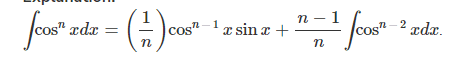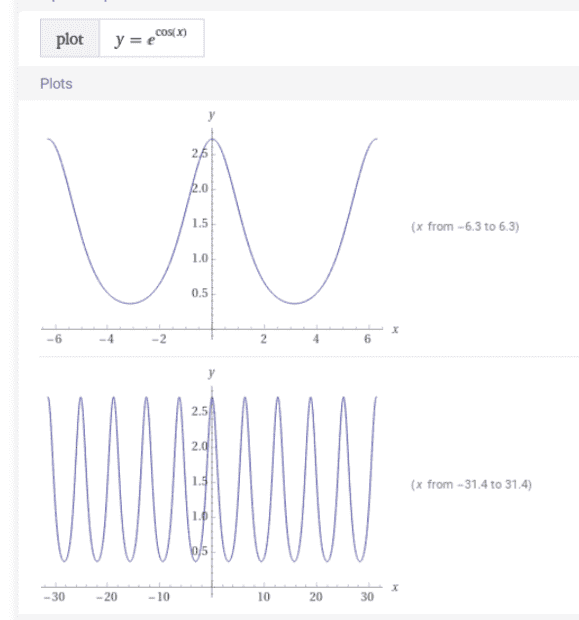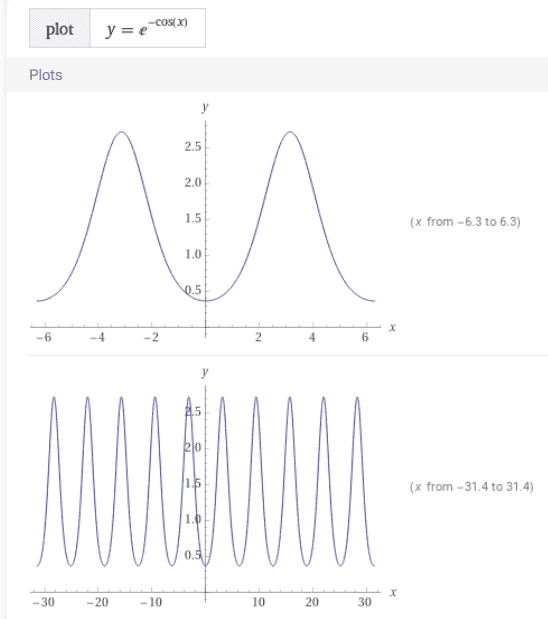# Can the integral of ##e^{\cos x}dx## be expressed as a unique Function?

Gold Member
Homework Statement:
Find the indefinite integral of ##e^{\cos x}dx##
Relevant Equations:
integration
I just came across this and it seems we do not have a definite answer...there are those who have attempted using integration by parts; see link below...i am aware that ##\cos x## has no closed form...same applies to the exponential function.

https://math.stackexchange.com/questions/2468863/what-is-the-integral-of-e-cos-x

would appreciate insight...

cheers!

Gold Member
y=e^(cos x) is a periodic function as we see in https://www.wolframalpha.com/input?i=graph+of+y＝e^(cos+x) .
$$\int_0^{2\pi} e^{\cos x}dx := 2\pi a \approx 7.95493$$
$$\int_0^{2\pi} (e^{\cos x}-a) dx = 0$$
This modified integral is periodic, i.e.,
$$\int_0^{X_1} (e^{\cos x}-a) dx = \int_0^{X_2} (e^{\cos x}-a) dx$$
where
$$X_2=X_1-2n\pi ,0<X_2<2\pi$$
Any of the modified integral is reduced to the integral in region ##[0,2\pi]##. Surely there exists the integral but I do not expect that it can be expressed by ordinary functions.

$$\int_{X_1}^{X_2} e^{\cos x}dx=I(x_2)-I(x_1)+a(X_2-X_1)$$
where
$$x_1=X_1-2n_1\pi, 0<x_1<2\pi$$
$$x_2=X_2-2n_2\pi, 0<x_2<2\pi$$
$$I(x):=\int _0^x (e^{\cos t}-a)dt,\ 0<x<2\pi$$

Last edited:
•chwala and topsquark
Homework Helper
2022 Award
I will amend the question my bad ; didn't get the English correctly...I meant being expressed as a function.

I think perhaps you are looking for "expressed in terms of elementary functions"; it is trivial, but uninformative, to define $$F(t) = \int_0^t e^{\cos u}\,du.$$

•chwala
Gold Member
I think you can try to have a infinite sum expanding by Taylor the exponential:

## \int e^{\cos(x)}dx=\int 1+\cos{x}+\frac{\cos^2{x}}{2!}+\frac{\cos^3{x}}{3!} dx ##

now by linearity:

## \int e^{\cos(x)}dx=x+\sin{x}+\int\frac{\cos^2{x}}{2!} dx+\int \frac{\cos^3{x}}{3!} dx + ...##

If you have a closed form for ##\int \cos^n{x}dx## I think you can find an expansion for ##\int e^{\cos{x}}dx##.

Ssnow

•anuttarasammyak and chwala
Gold Member
I think you can try to have a infinite sum expanding by Taylor the exponential:

∫ecos⁡(x)dx=∫1+cos⁡x+cos2⁡x2!+cos3⁡x3!dx

now by linearity:

∫ecos⁡(x)dx=x+sin⁡x+∫cos2⁡x2!dx+∫cos3⁡x3!dx+...

If you have a closed form for ∫cosn⁡xdx I think you can find an expansion for ∫ecos⁡xdx.

Ssnow
we may do further reduction making use offrom https://socratic.org/questions/how-do-you-find-the-integral-of-cos-n-x .
I am not patient and see it messy.

Last edited:
•chwala
Gold Member
interesting ...how would we attempt to find then the limits of ##e^{-\cos x}## and ##e^{cos x}## as ##x## tends to infinity? my interest is on the approach, i can tell from the graph that the limits tend to ##±∞##.

Gold Member
They are periodical functions as well as cos x is. They have no limits for x=##\pm \infty##.$$e^{-\cos x}=e^{\cos(x+\pi)}$$Last edited:
Gold Member
They are periodical functions as well as cos x is. They have no limits for x=##\pm \infty##.View attachment 322205

$$e^{-\cos x}=e^{\cos(x+\pi)}$$
View attachment 322206
I need to look at this, can we say that it is bounded ...supremum, infimum? need to check on this man!

Gold Member
$$e^{-1} \le e^{\cos x} \le e$$

•chwala
Gold Member
$$e^{-1} \le e^{\cos x} \le e$$
Nice, yes, If ##y=\cos x## then we have the maximum at ##y=1## and minimum at ##y=-1##... then how comes that this function has no limit? given
##\lim_{x \rightarrow +\infty} {e^{cos x}}##

am i missing something here...

•